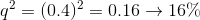# MCAT Biology : Other Evolution Principles

## Example Questions

### Example Question #11 : Evolution

Inbreeding reduces the fitness of a population. This is the result of which increased genetic effect of inbreeding?

Expression of deleterious recessive traits

Levels of aggression

Genetic diversity

Rate of spontaneous mutation

Expression of deleterious recessive traits

Explanation:

Inbreeding increases the expression of recessive traits due to more heterozygous carriers mating with each other. As the same individuals mate, the chance of a homozygous recessive child increases. This is the same as estimating the likelihood of a single healthy child from two carrier parents (0.75) versus eight healthy children from two carrier parents (0.10).

Inbreeding decreases genetic diversity, rather than increasing it. The rate of spontaneous mutation is not impacted by this type of breeding. There is no reason to infer increased levels of aggression.

### Example Question #1 : Understanding Hardy Weinberg Equilibrium

Which is not a necessary condition for the Hardy-Weinberg equation to be true?

No natural selection

No net migration of individuals into or out of the population

No mutations in the gene pool

Random mating

Small population

Small population

Explanation:

For the Hardy-Weinberg equation to be true, the population in question must be very large. This ensures that coincidental occurrences do not drastically alter allelic frequencies.

### Example Question #10 : Evolution And Mutations

In a population that is in Hardy-Weinberg equilibrium, the frequency of homozygous dominant individuals is 0.36. What is the percentage of homozygous recessive individuals in the population?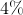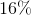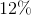Explanation:

The two equations pertaining to Hardy-Weinberg equilibrium are: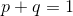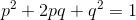In this second equation, each term refers to the frequency of a given genotype.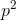is the homozygous dominant frequency,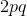is the heterozygous frequency, and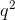is the homozygous recessive frequency.

From the question, we know that: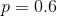We now know the dominant allele frequency. Using the other Hardy-Weinberg equation, we can find the recessive allele frequency: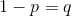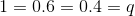Returning to our genotype frequency terms, we can use this recessive allele frequency to find the homozygous recessive frequency: